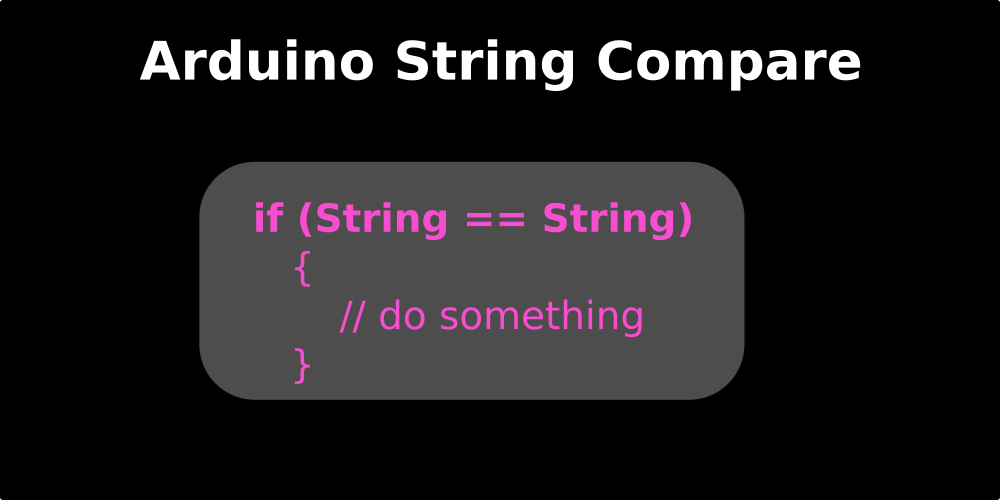Arduino Compare Strings From Serial Monitor

Compare Strings Arduino

String Arduino Compare How to Compare Strings from Serial Communication - String Language Simple is a sentence. Comparing strings on Arduino programming is not as easy as comparing numbers such as int and float.How to compare Arduino strings

In comparing the string or string of Arduino Compare, there are several methods used, depending on where our strings you want to compare.

A. Operator for Compare a String

To be able to compare a value, we need a comparison operator. Likewise with strings. String comparison operators include:

• "==" read "the same as"
• "! =" read "not the same as"
•   "<" read "is smaller than"
•   "> =" read "greater the same as"
•   "<=" read "smaller the same as"
• "Equals ()" is the same as the operator "=="

This command:
if (stringFirst == stringSecond) {
Equals with:
if (stringFirst.equals(stringSecond)) {

B. Program comparing basic Arduino strings

The following is the code for the predetermined string example. We will compare two string values.

We make stringFirst = "test" and stringSecond = "test". If these two strings are true and the same, then Arduino will send notifications to the monitor series with the word "this word is the same".

Here is the program:

String stringFirst = "tes";
String stringSecond = "tes";

void setup() {
Serial.begin(9600);
}

void loop() {
if (stringFirst == stringSecond)
{
Serial.println("yes");
}

else Serial.println("not");
delay(5000);
}

Please open the Arduino Monitor series, then the statement will appear. Now, please change the word "test" from one string into another word. Then upload the program again.

Look back at the Arduino Monitor series, you will see the results of his statement.

C. The program compares Arduino strings from serial communication

String handling on data from this series is different from the set data. To read string data from the serial and compare it, there are two methods, namely:

1. Read the string from the serial to the data '\ n', then the data is cut and then compared.
2. Read the string from the serial to the data '\ n', then the data is changed directly to the char array, then compared

Now we will make a simple Arduino project, turn on the LED through the string communication series.

The example of the project to be used is, turning on 3 LEDs with different colors with the string command.

• String "red on" to turn on the red light, "red off" to turn it off.
• String "green on" to turn on the green light, "green off" to turn it off.
• String "blue on" to turn on the blue light, "Blue Off" to turn it off.
Before starting the program, the circuit we will use is like this:LED testing circuit through Arduino series (Blue = Biru, Green = Hijau, and Red = Merah)

1. Read string data to contain '\ n' data then cut the data

Now we will learn how to compare the string values sent through serials.

/* www.chippiko.com

Program Membandingkan String Dari Serial
Untuk menghidupkan LED
*/

int ledMerah = 13;
int ledHijau = 12;
int ledBiru  = 11;

void setup() {
Serial.begin(9600);

pinMode(ledMerah, OUTPUT);
pinMode(ledHijau, OUTPUT);
pinMode(ledBiru, OUTPUT);
}

void loop() {
while (Serial.available() > 0) {
delay(100);

if (read_string == "Merah On") {
digitalWrite(ledMerah, HIGH);
}

if (read_string == "Merah Off") {
digitalWrite(ledMerah, LOW);
}

if (read_string == "Hijau On") {
digitalWrite(ledHijau, HIGH);
}

if (read_string == "Hijau Off") {
digitalWrite(ledHijau, LOW);
}

if (read_string == "Biru On") {
digitalWrite(ledBiru, HIGH);
}

if (read_string == "Biru Off") {
digitalWrite(ledBiru, LOW);
}
}
}

After the program is uploaded, open the monitor series. Type the string, for example "Merah On" then send. Notice on the LED whether the lights are on. If you don't check the circuit.

2. Change String Data to Char Array

Now, with the same project, we will first change the string received to be char array. This is actually a better way.

/* www.chippiko.com

Program Membandingkan String Dari Serial
Untuk menghidupkan LED
*/

int ledMerah = 13;
int ledHijau = 12;
int ledBiru  = 11;

char bufferString;

void setup() {
Serial.begin(9600);

pinMode(ledMerah, OUTPUT);
pinMode(ledHijau, OUTPUT);
pinMode(ledBiru, OUTPUT);
}

void loop() {
while (Serial.available() > 0) {

if (strcmp(bufferString, "Merah On") == 0) {
Serial.println(bufferString);
digitalWrite(ledMerah, HIGH);
}
if (strcmp(bufferString, "Merah Off") == 0) {
Serial.println(bufferString);
digitalWrite(ledMerah, LOW);
}

if (strcmp(bufferString, "Hijau On") == 0) {
digitalWrite(ledHijau, HIGH);
}
if (strcmp(bufferString, "Hijau Off") == 0) {
digitalWrite(ledHijau, LOW);
}

if (strcmp(bufferString, "Biru On") == 0) {
digitalWrite(ledBiru, HIGH);
}
if (strcmp(bufferString, "Biru Off") == 0) {
digitalWrite(ledBiru, LOW);
}
memset(bufferString, 0, sizeof(bufferString));
}
}

I hope this Arduino Compare string article can help you. Thank you for visiting the Chip Piko website.

Hopefully useful.

Sumber :